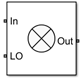# IMT Mixer

Model mixer using intermodulation table (IMT)

• Library:
• RF Blockset / Circuit Envelope / Elements

•## Description

Use the IMT Mixer to perform frequency translation defined in an intermodulation table (see , , , and ) for a single tone carrier mixed with a local oscillator (LO) signal. The block includes nonlinear amplification, device and phase noise, and mixer spur visualization. For a single tone carrier Fcar nonlinearly modulated with an LO signal of frequency FLO, the mixer output intermodulation products occur at frequencies:

`${F}_{\text{out}}\left(M,N\right)=|M×{F}_{\text{car}}±N×{F}_{\text{LO}}|$`

where:

• Fcar – input RF signal carrier frequency

• FLO – local oscillator frequency

• M and N are nonnegative integers (0,1,…, order of nonlinearity)

For a downconverter, the desired output tone is $|{F}_{\text{car}}-{F}_{\text{LO}}|$, and for an upconverter it is $|{F}_{\text{car}}+{F}_{\text{LO}}|$. All other combinations of M and N represent the spurious intermodulation products.

## Parameters

expand all

Main

Carrier frequency, specified as a scalar in hertz. When multiple carriers exist on the input connection, this carrier frequency is selected as the RF signal input. Distance between adjacent carriers must be greater than $\left(1-1{e}^{-8}\right){F}_{\text{c}}$.

Data Types: `double`

Local oscillator (LO) frequency, specified as a scalar in hertz.

Reference input power, specified as a scalar in dBm. The expression for the normalized input signal for the specified reference input power is:

`${10}^{\left(\frac{\left(-{P}_{\text{rf}}+20\mathrm{log}10\left(\frac{1}{\sqrt{{R}_{\text{in}}}}\right)+30\right)}{20}\right)}$`

Nominal output power, specified as a scalar in dBm. The expression for the normalized output signal for the specified reference input power is:

`$2•{10}^{\left(\frac{\left({P}_{\text{if}}-20\mathrm{log}10\left(\frac{1}{\sqrt{{R}_{\text{out}}}}\right)-30\right)}{20}\right)}$`

Select this parameter to specify the data file you want to use to extract the spur table. Clear to specify your own spur values. The data file may contain any combination of IMT table and colored spot noise in s2d or p2d format. See .

Data file, specified as IMT data and colored spot noise.

#### Dependencies

To set this parameter, first select Use data file.

Input impedance of mixer, specified as a real scalar.

Output impedance of mixer, specified as a real scalar.

Select this parameter to internally ground and hide the negative terminals. To expose the negative terminals, clear this parameter. If the terminals are exposed, the input signal is not referenced to the ground.

IMT

IMT spur visualization, specified as a square matrix.

Signal power of the desired output tone when plotting intermodulation products, specified as a scalar.

Mixer type, specified as `Upconverter` or `Downconverter`.

Visualize IMT table values using specified signal power and mixer type.

Noise

Select this parameter to simulate noise as specified in block parameters or in file.

If the noise is specified in an `.s2p` file, then it is used for simulation.

Noise type, specified as `Noise figure` or `Spot noise data`.

#### Dependencies

To set this parameter, first select Simulate noise.

Noise distribution, specified as:

• `White` – Spectral density is a single nonnegative value. The power value of the noise depends on the bandwidth of the carrier, and the bandwidth depends on the time step. This is an uncorrelated noise source.

• `Piece-wise linear` – Spectral density is a vector of values [pi]. For each carrier, the noise source behaves like a white uncorrelated noise. The power of the noise source is carrier dependent.

• `Colored` – Depends on both carrier and bandwidth. This is a correlated noise source.

#### Dependencies

To set this parameter, first select Simulate noise.

Noise figure, specified as a scalar in decibels.

#### Dependencies

To set this parameter, first select Simulate noise.

Frequency data, specified as a scalar for white noise or vector for piece-wise linear or colored noise in hertz.

#### Dependencies

To set this parameter, first select Select noise then select `Piece-wise linear` or `Colored` in Noise distribution.

Minimum noise figure, specified as a scalar or vector in decibels.

#### Dependencies

To set this parameter, first select ```Spot noise data``` in Noise type.

#### Dependencies

To set this parameter, first select Select noise then select `Spot noise data` in Noise Type.

Optimal reflection coefficient, specified as a scalar or a vector.

#### Dependencies

To set this parameter, first select Select noise then select `Spot noise data` in Noise Type.

Equivalent normalized noise resistance, specified as a scalar or vector.

#### Dependencies

To set this parameter, first select Select noise then select `Spot noise data` in Noise Type.

Select this parameter to add phase noise to your system with a continuous wave source.

#### Dependencies

To set this parameter, select Simulate noise.

Phase noise frequency offset with respect to LO signal, specified as a scalar or vector with each element unit in hertz.

The frequency offset values must be bounded by the envelope bandwidth of the simulation. For more information see Configuration.

#### Dependencies

To enable this parameter, first select Simulate noise then select Add phase noise.

Phase noise level, specified as a scalar or vector or matrix with elements in decibel per hertz.

If you specify a matrix, each column should correspond to a non-DC carrier frequency of the CW source. The frequency offset values must be bounded by the envelope bandwidth of the simulation. For more information see Configuration.

#### Dependencies

To enable this parameter, first select Simulate noise then select Add phase noise.

Select this parameter to automatically calculate impulse response for frequency-dependent noises. Clear this parameter to manually specify the impulse response duration using Impulse response duration.

#### Dependencies

To enable this parameter, first select Simulate noise then select Add phase noise.

Impulse response duration used to simulate frequency-dependent noise, specified as a scalar in seconds. The time should be an integer multiple of the step size in the configuration block, ```Tduration = NTstep```.

#### Dependencies

To set this parameter, first clear Automatically estimate impulse response duration.

 https://www.mathworks.com/help/rf/examples/visualizing-mixer-spurs.html

 https://www.microwavejournal.com/articles/3430-the-use-of-intermodulation-tables-for-mixer-simulations